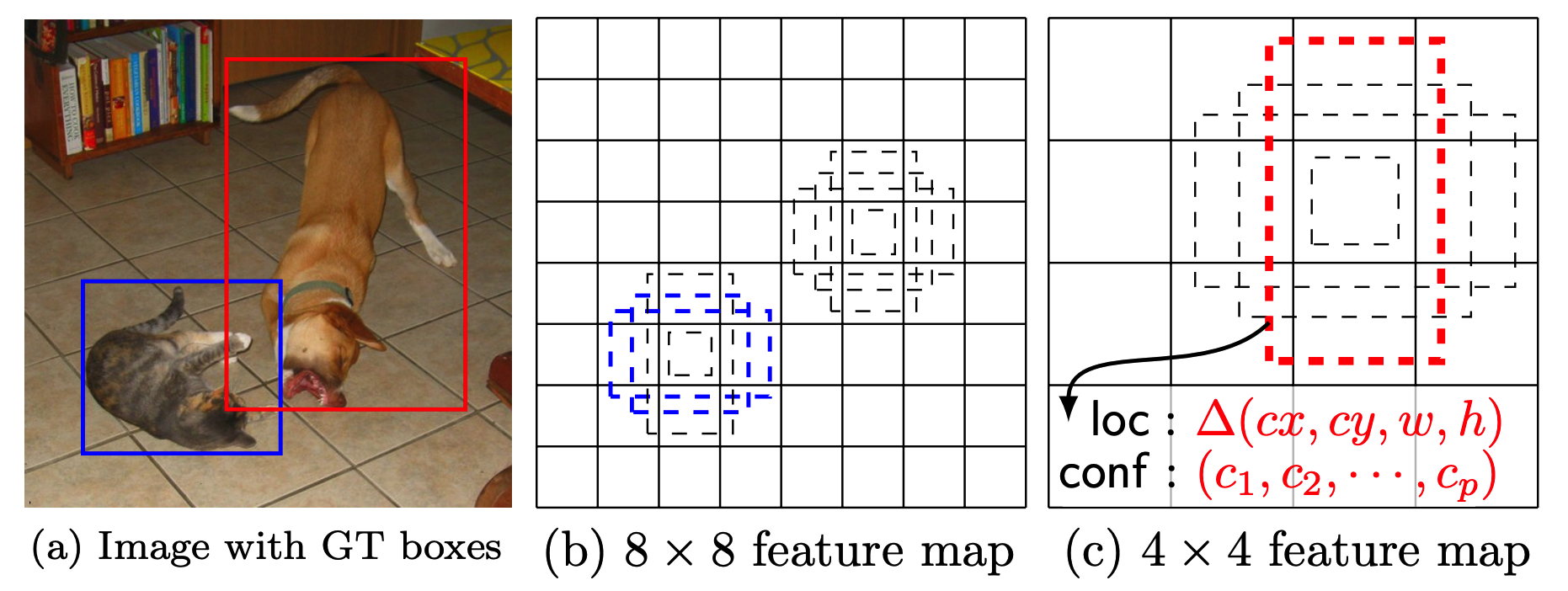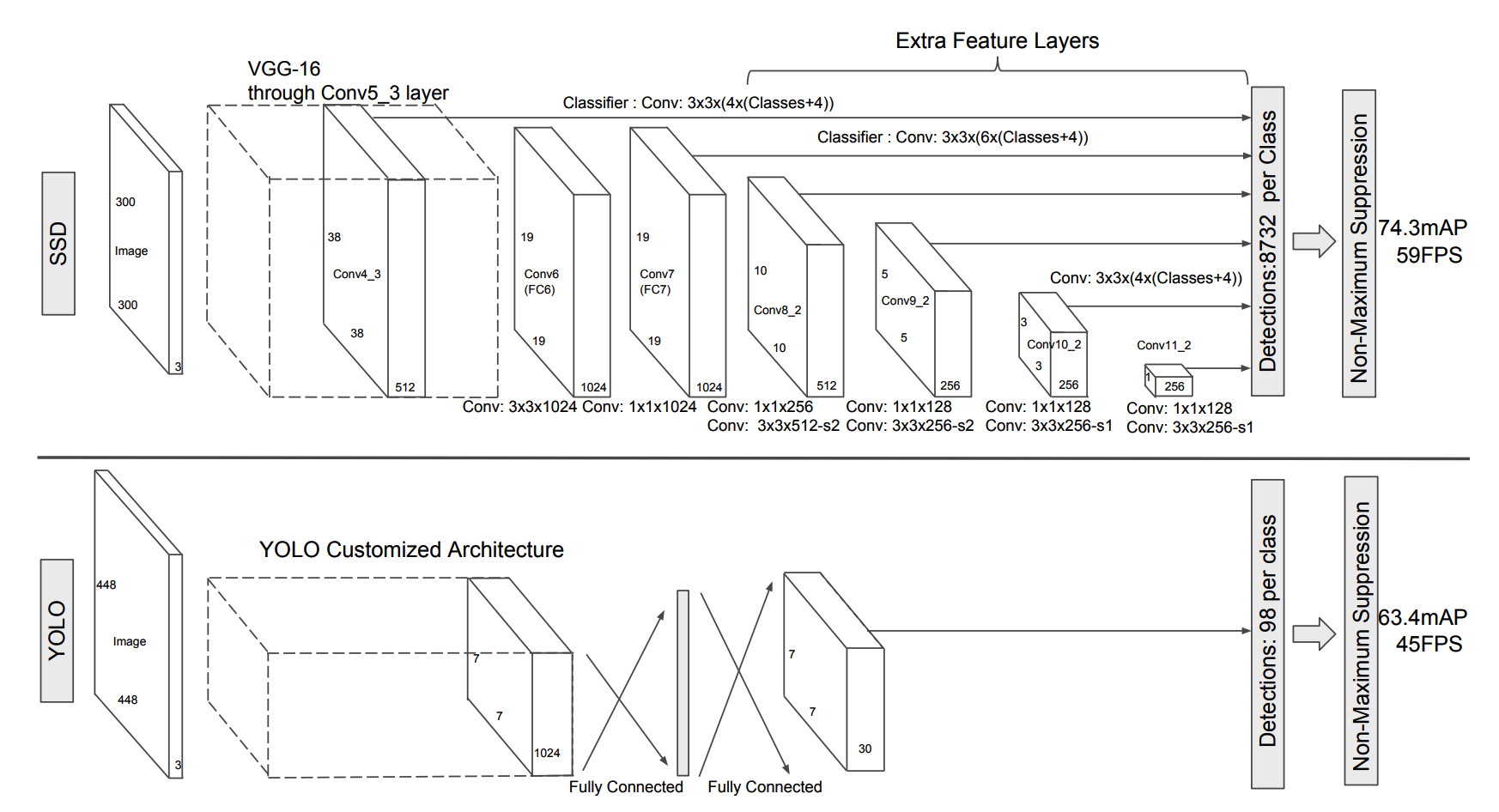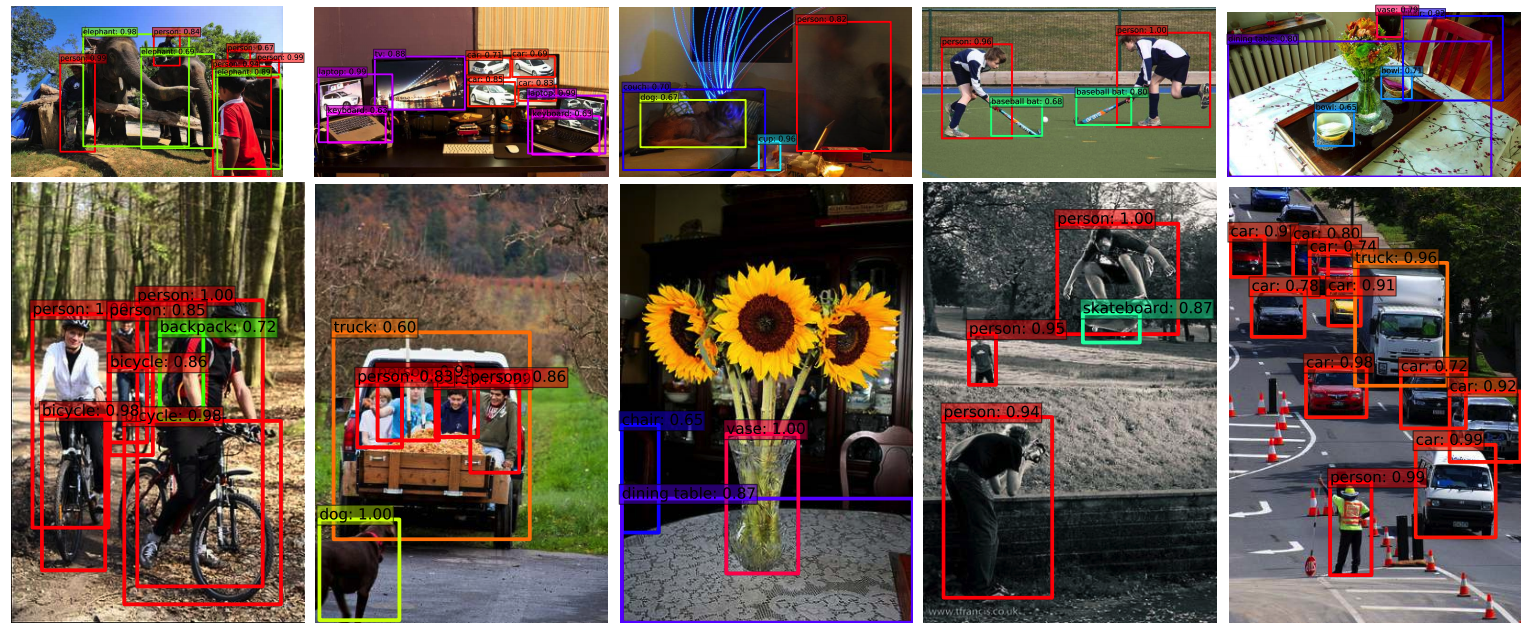# SSD: Single Shot MultiBox Detector

## WHY?

Object box proposal process is complicated and slow in object detection process. This paper proposes Single Shot Detector(SSD) to detect objects with single neural network.

## WHAT?SSD prodeces fixed-size collection of bounding boxes and scores the presence of class objects in the boxes.The front of SSD is standard classification model with truncated classification layer(base network). After the base network, convolution layers added to decrease the size progressively. These progressively decreasing feature layers represent multiple granuality of bounding boxes. For each feature map, multiple scale(k) classifiers are applied to 3 x 3 area to compute scores of c class labels and 4 relative offsets. For m x n feature map, 3 x 3 filter with k x (c + 4) channel classfiers are applied to produce (c + 4)kmn outputs.

This process require ground truth bounding boxes. Default boxes which has higher jaccard overlap with ground truth boxes than a threshold(0.5) are considered as answer. The training objective consists of two parts: the localization loss and the confidence loss. with x_{ij}^k$x_{ij}^k$ indicates the matching the i-th default box to the j-th ground truth box of category p.

L(x, c, l, g) = \frac{1}{N}(L_{conf}(x,c) + \alpha L_{loc}(x, l, g))\\
L_{loc}(x, l, g) = \sum^N_{i\in Pos}\sum_{m\in\{cx, cy, w, h\}} x_{ij}^k smooth_{L1}(l_i^m - \hat{g}_j^m)\\
\hat{g}_j^{cx} = \frac{(g_j^{cx} - d_i^{cx})}{d_i^w}\\
\hat{g}_j^{cy} = \frac{(g_j^{cy} - d_i^{cy})}{d_i^h}\\
\hat{g}_j^{w} = \log \frac{g_j^{w}}{d_i^w}\\
\hat{g}_j^{h} = \log \frac{g_j^{h}}{d_i^h}\\
L_{conf}(x, c) = - \sum^N_{i\in Pos}x_{ij}^p \log(\hat{c}_i^p) - \sum_{i\in Neg}\log(\hat{c}_i^0)

Boxes of different aspect ratios are proposed for each feature map.

s_k = s_{min} + \frac{s_{max} - s_{min}}{m - 1}(k-1), k\in[1,m]

With feature map of differnt granuality and aspect ratios, SSD can a wide range of boxes.

## So?SSD achieved better result on PASCAL VOC2007, 2012 and COCO than Fast and Faster R-CNN.

## Critic

Integrating redundent and slow process into neural network seems convenient idea. However, I think there would be better way to suggest boxes than proposing hundreds of them.

Powered by aiden